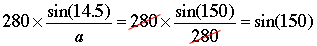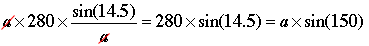SEARCH HOMEMath Central Quandaries & QueriesQuestion from ken, a student: how do you do the steps to this equation. sin14.5/a = sin150/280 the text book answer is 140.21 miles ,but how do the steps?Hi Ken,

If you multiply both sides of the equation by 280 you can cancel 280 from the numerator and denominator on the right to get.

Now multiply each side by a and again cancel to get.

Evaluate sin(14.5o) and sin(150o) in the expression

280 × sin(14.5) = a × sin(150)

and solve for a.

Write back if you need more help,
PennyMath Central is supported by the University of Regina and The Pacific Institute for the Mathematical Sciences.